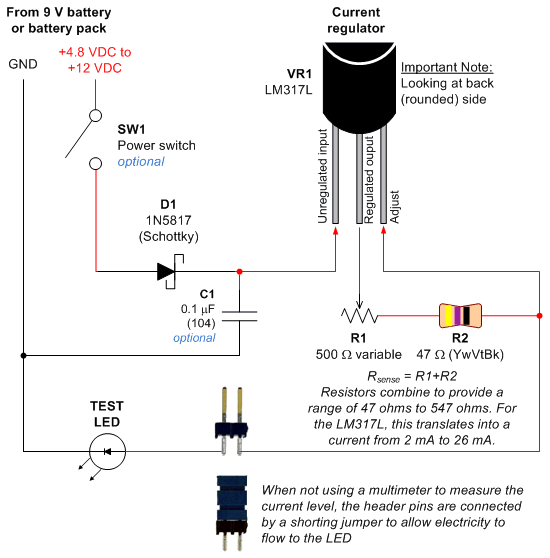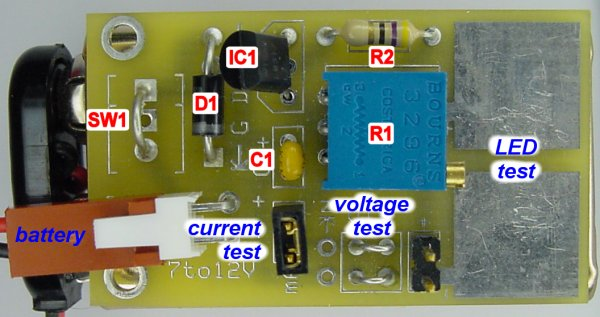• Home• Patreon• Links• Books
LED Tester:

# 3. Schematic and Circuit Board for LED Test Equipment

(article continued from previous page)

Most of my circuits still use 5 V regulated power for the chips, because 8-bit microcontrollers run faster and some sensors require 5 V. Since 5 V is more than enough for even a white LED, I’m not concerned with the voltage needed by a particular LED in use.

However, since my robots are battery powered, I am concerned with the current usage, since that determines how quickly the battery is exhausted. Furthermore, an LED can be destroyed by too much current (usually anything beyond 30 mA). Therefore, I decided to make the LED tester based on current, not voltage.

As described previously, the LED tester can be set to supply a specific current from 2 mA to 26 mA. The circuit will automatically vary the voltage from nearly 0 V to about 7.5 volts in order to supply the current. I don’t need to adjust the voltage, and I don’t need to care about the current after I’ve set it.

Many people are accustomed to voltage regulators, like the standard 7805. But, surprisingly, most voltage regulators can be set up to regulate current instead.

For this circuit, I chose the National Semiconductor LM317L that I purchased from DigiKey or Mouser Electronics (or LM317LZ from Electronic Goldmine). The LM317L is cheap, small, and includes instructions in the datasheet for using it as a current regulator. The semiconductor circuitry inside of the LM317L uses a simple feedback loop to automatically vary the output voltage to meet the required current.Schematic of an LED test circuit that supplies constant current.

SW1: (optional) The power switch is optional in this circuit because current can only pass through a test LED and the capacitor (C1). Low value ceramic capacitors at low voltages leak very little current (less than 1/10 of a nanoamp as far as I could measure). And, there’s no point of connecting a test LED with the power off. Since no power will be used when idle, the power switch is unnecessary.

D1: The 1N5817 Schottky diode prevents current from flowing backwards if the battery is connected backwards. This protects the LM317L against damage.

C1: (optional) Most regulators and integrated circuits achieve stability from the addition of capacitors. There’s nothing critical going on in this circuit (no computations, high currents, current spikes, or high voltages). So, this capacitor is probably unnecessary.

VR1: The LM317L voltage regulator is the core of this circuit.

The test LED (or any other load) draws power from output pin through R1+R2. The adjust pin measures the voltage just after R1+R2. By subtracting the adjust voltage from the output voltage, the LM317L is able to measure exactly how much voltage is dropped across R1+R2.

Because all current that passes through the LED must first pass through R1+R2, the current must be the same for the LED and R1+R2. According to Ohm’s law, the current that passes through a fixed resistor results in a proportional voltage drop. Therefore, the more current drawn by the LED, the more current passes through R1+R2, the greater the voltage drop across R1+R2. The less current drawn by the LED, the less current passes through R1+R2, the lower the voltage drop across R1+R2.

So, the LM317L is able to regulate the total current delivered to the LED by constantly measuring the voltage dropped across the sense resistor (R1+R2) and then lowering or raising the voltage at the output pin accordingly.

By the way, a fixed resistor configured to measure current by measuring voltage drop is called a “sense” resistor. For example, a small resistor (less than 1 ohm) is often placed in series with a motor driver circuit to detect how much current a motor is drawing.

R1: The potentiometer can change resistance from 0 ohms to 500 ohms. As previously discussed, this alters the output voltage of the LM317L to allow you to adjust the current provided.

R2: This fixed resistor provides a maximum current limit of 26 mA based on the LM317L datasheet’s formula. If this resistor wasn’t included in the circuit and the potentiometer was dialed to 0 ohms, the test LED would receive significantly more current from the LM317L (perhaps as much as 300 mA), damaging the LED. So, this resistor exists to protect the LED.

The formula for computing the amount of current supplied by the LM317L is:

`(1.2 V / (R1 + R2)) * 1000 = current in milliamps`
`Maximum: (1.2 V / (0 ohms + 47 ohms)) * 1000 = 25.5 mA`
`Minimum: (1.2 V / (500 ohms + 47 ohms)) * 1000 = 2.2 mA`LED tester PCB labeled with component identifiers.

You could actually use this circuit in a robot charging station. It would limit the maximum amount of current provided to the robot’s batteries. Also, if the robot (or something from the environment) accidentally shorted out the charging station contacts, the maximum current would be limited.

## Update!

Now there is a version of this LED test tool with an LCD display for showing the current, voltage drop, and recommended resistor. Also, a video!